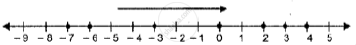# In the Following Case, Arrange the Given Integers in Ascending Order Using a Number Line. 3, – 3, 4, – 7, 0, – 6, 2 - Mathematics

Sum

In the following case, arrange the given integers in ascending order using a number line.

3, – 3, 4, – 7, 0, – 6, 2

#### Solution

3, -3, 4, -7, 0, -6, 2
Draw the number line and mark the numbers on it. Arranging in ascending order as shown on the number line. -7, -6, -3, 0, 2, 3, 4Concept: Operation on of Whole Number on Number Line
Is there an error in this question or solution?

#### APPEARS IN

Selina Class 6 Mathematics
Chapter 7 Number Line
Exercise 7 (A) | Q 5.2